## Friday, January 25, 2019

### In Vivo Magnetic Recording of Neuronal Activity

In Section 8.9 of Intermediate Physics for Medicine and Biology, Russ Hobbie and I discuss the detection of weak magnetic fields produced by the body.
The detection of weak fields from the body is a technological triumph. The field strength from lung particles is about 10-9 T [Tesla]; from the heart it is about 10-10 T; from the brain it is 10-12 T for spontaneous (α-wave) activity and 10-13 T for evoked responses. These signals must be compared to 10-4 T for the earth’s magnetic field. Noise due to spontaneous changes in the earth’s field can be as high as 10-7 T. Noise due to power lines, machinery, and the like can be 10-5–10-4 T.
This triumph was possible because of ultra-sensitive superconducting quantum interference device (SQUID) magnetometers. These magnetometers, however, operate at cryogenic temperatures and therefore must be used outside the body. For instance, to measure the magnetic field of the brain (the magnetoencephalogram), pickup coils must be at least several centimeters from the neurons producing the biomagnetic field because of the thickness of the scalp and skull. A great advantage of SQUIDs is they are completely noninvasive. Yet, when the magnetic field is measured far from the source reconstructing the current distribution is difficult.

Imagine what you could do with a really small magnetometer, say one you could put into the tip of a hypodermic needle. At the cost of being slightly invasive, such a device could measure the magnetic field inside the body right next to its source. The magnetic fields would be larger there and you could get exquisite spatial resolution.

Last September, Laure Caruso and her coworkers published an article about “In Vivo Magnetic Recording of Neuronal Activity” (Neuron, Volume 95, Pages 1283–1291, 2017).
Abstract: Neuronal activity generates ionic flows and thereby both magnetic fields and electric potential differences, i.e., voltages. Voltage measurements are widely used but suffer from isolating and smearing properties of tissue between source and sensor, are blind to ionic flow direction, and reflect the difference between two electrodes, complicating interpretation. Magnetic field measurements could overcome these limitations but have been essentially limited to magnetoencephalography (MEG), using centimeter-sized, helium-cooled extracranial sensors. Here, we report on in vivo magnetic recordings of neuronal activity from visual cortex of cats with magnetrodes , specially developed needle-shaped probes carrying micron-sized, non-cooled magnetic sensors based on spin electronics. Event-related magnetic fields inside the neuropil were on the order of several nanoteslas, informing MEG source models and efforts for magnetic field measurements through MRI. Though the signal-to-noise ratio is still inferior to electrophysiology, this proof of concept demonstrates the potential to exploit the fundamental advantages of magnetophysiology.A Magnetrode. Adapted from Fig. 1a in Caruso et al. (2017) Neuron 95:1283–1291.
The measurements are made using giant magnetoresistance sensors: a magnetic-field dependent resistor. The size of the sensor was roughly 50 by 50 microns, and was etched to have the shape of a needle with a sharp tip. It can detect magnetic fields of a few nanoTesla (10-9 T). To test the system, Caruso and her colleagues measured evoked fields in a cat's visual cortex. Remarkably, they performed these experiments with no shielding whatsoever (SQUIDs often require bulky and expensive magnetic shields). When they recorded the magnetic field without averaging it was noisy, so most of their data is after 1000 averages. They removed 50 Hz power line contamination by filtering, and they could distinguish direct magnetic field coupling from capacitive coupling.

When I was in graduate school, John Wikswo and I measured the magnetic field of a single axon using a wire-wound toroidal core. We were able to measure 0.2 nT magnetic fields without averaging and with a signal-to-noise ratio over ten. However, our toroids had a size of a few millimeters, which is larger than Caruso et al.’s magnetrodes. Both methods are invasive, but John and I had to thread the nerve through the toroid, which I think is more invasive than poking the tissue with a needle-like probe.

A couple years ago in this blog, I discussed a way to measure small magnetic fields using optically probed nitrogen-vacancy quantum defects in diamond. That technique has a similar sensitivity as magnetrodes based on giant magnetoresistance, but the recording device is larger and requires an optical readout, which seems to me more complicated than just measuring resistance.

My favorite way to detect fields of the brain would be to use the biomagnetic field as the gradient in magnetic resonance imaging. This method would be completely noninvasive, could be superimposed directly on a traditional magnetic resonance image, and would measure the magnetic field in every pixel simultaneously. Unfortunately, such measurements are barely possible after much averaging even under the most favorable conditions.

Caruso et al. speculate about using implantable magnetrodes with no connecting wires.
Implanted recording probes play an important role in many neurotechnological scenarios. Untethered probes are particularly intriguing, as they avoid connection wires and corresponding limitations.
The recording of tiny biomagnetic fields seems to be undergoing a renaissance, as new detectors are developed. It is truly a technological triumph.

## Friday, January 18, 2019

### Five New Homework Problems About Diffusion

Diffusion is a central concept in biological physics, but it's seldom taught in physics classes. Russ Hobbie and I cover diffusion in Chapter 4 of Intermediate Physics for Medicine and Biology.

The one-dimensional diffusion equation,
is one of the “big threepartial differential equations. Few analytical solutions to this equation exist. The best known is the decaying Gaussian (Eq. 4.25 in IPMB). Another corresponds to when the concentration is initially constant for negative values of x and is zero for positive values of x (Eq. 4.75). This solution is written in terms of error functions, which are integrals of the Gaussian (Eq. 4.74). I wonder: are there other simple examples illustrating diffusion? Yes!

In this post, my goal is to present several new homework problems that provide a short course in the mathematics of diffusion. Some extend the solutions already included in IPMB, and some illustrate additional solutions. After reading each new problem, stop and try to solve it!

Section 4.13
Problem 48.1. Consider one-dimensional diffusion, starting with an initial concentration of C(x,0)=Co for x less than 0 and C(x,0)=0 for x greater than 0. The solution is given by Eq. 4.75
where erf is the error function.
(a) Show that for all times the concentration at x=0 is C0/2.
(b) Derive an expression for the flux density, j = -DC/∂x at x = 0. Plot j as a function of time. Interpret what this equation is saying physically. Note:

Problem 48.2. Consider one-dimensional diffusion starting with an initial concentration of C(x,0)=Co for |x| less than L and 0 for |x| greater than L.
(a) Plot C(x,0), analogous to Fig. 4.20.
(b) Show that the solution
obeys both the diffusion equation and the initial condition.
(c) Sketch a plot of C(x,t) versus x for several times, analogous to Fig. 4.22.
(d) Derive an expression for how the concentration at the center changes with time, C(0,t). Plot it.

Problem 48.3. Consider one-dimensional diffusion in the region of x between -L and L. The concentration is zero at the ends, CL,t)=0.
(a) If the initial concentration is constant, C(x,0)=Co, this problem cannot be solved in closed form and requires Fourier series introduced in Chapter 11. However, often such a problem can be simplified using dimensionless variables. Define X = x/L, T = t/(L2/D) and Y = C/Co. Write the diffusion equation, initial condition, and boundary conditions in terms of these dimensionless variables.
(b) Using these dimensionless variables, consider a different initial concentration Y(X,0)=cos(Xπ/2). This problem has an analytical solution (see Problem 25). Show that Y(X,T)=cos(Xπ/2) e2T/4 obeys the diffusion equation as well as the boundary and initial conditions.

Problem 48.4. In spherical coordinates, the diffusion equation (when the concentration depends only on the radial coordinate r) is (Appendix L)

Let C(r,t) = u(r,t)/r. Determine a partial differential equation governing u(r,t). Explain how you can find solutions in spherical coordinates from solutions of analogous one-dimensional problems in Cartesian coordinates.

Problem 48.5. Consider diffusion in one-dimension from x = 0 to ∞. At the origin the concentration oscillates with angular frequency ω, C(0,t) = Co sin(ωt).
(a) Determine the value of λ that ensures the expression
obeys the diffusion equation.
(b) Show that the solution in part (a) obeys the boundary condition at x = 0.
(c) Use a trigonometric identity to write the solution as the product of a decaying exponential and a traveling wave (see Section 13.2). Determine the wave speed.
(d) Plot C(x,t) as a function of x at times t = 0, π/2ω, π/ω, 3π/2ω, and 2π/ω.
(e) Describe in words how this solution behaves. How does it change as you increase the frequency?

Of the five problems, my favorite is the last one; be sure to try it. But all the problems provide valuable insight. That’s why we include problems in IPMB, and why you should do them. I have included the solutions to these problems at the bottom of this post (upside down, making it more difficult to check my solutions without you trying to solve the problems first).Random Walks in Biology,by Howard Berg.
Interested in learning more about diffusion? I suggest starting with Howard Berg’s book Random Walks in Biology. It is at a level similar to Intermediate Physics for Medicine and Biology.

After you have mastered it, move on to the classic texts by Crank (The Mathematics of Diffusion) and Carslaw and Jaeger (Conduction of Heat in Solids). These books are technical and contain little or no biology. Mathephobes may not care for them. But if you’re trying to solve a tricky diffusion problem, they are the place to go.

Enjoy!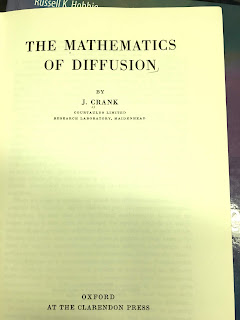The Mathematics of Diffusion.The Conduction of Heat in Solids.I told you these books are technical! (Page 45 of Crank)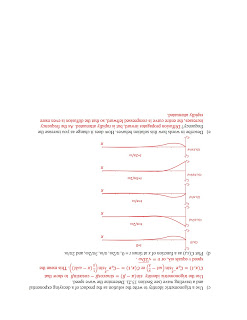Page 4Page 3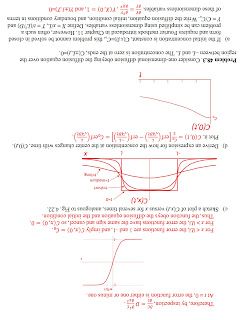Page 2Page 1

## Friday, January 11, 2019

Section 10.8 of Intermediate Physics for Medicine and Biology describes the radial isochron clock, a toy model for electrical stimulation of nerve or muscle. Russ Hobbie and I write:
Many of the important features of nonlinear systems do not occur with one degree of freedom. We can make a very simple model system that displays the properties of systems with two degrees of freedom by combining the logistic equation for variable r with an angle variable θ that increases at a constant rate:
We can interpret (r,θ) as the polar coordinates of a point in the xy plane. When [time] t has increased from 0 to 1 the angle has increased from 0 to 2π, which is equivalent to starting again with θ = 0. This model system has been used by many authors. Glass and Mackey (1988) have proposed that it be called the radial isochron clock.Fig. 10.19 of IPMB.
We use this model to analyze phase resetting. Let the clock run until it settles into a stable limit cycle, in which case the signal x(t) is a sinusoidal oscillation. Then apply a stimulus that suddenly increases x by an amount b (see Fig. 10.19 in IPMB) and observe the resulting dynamics. The system returns to its limit cycle, but with a different phase. The first plot in the figure below shows the period T/T0 of the oscillator just after a stimulus is applied at TS/T0; it's the same illustration as in Fig 10.20b of IPMB. Something dramatic appears to be happening at TS/T0 = 0.5. What's going on?The radial isochron clock for different stimulus times and strengths.
The problem with a plot of T/T0 versus TS/T0 is that I have difficulty relating it to the behavior of the signal as a function of time, x(t). Above I plot x versus t for four cases:
• TS/T0 = 0.25, b = 0.95 (blue dot). In this case, the stimulus is applied soon after the peak when the signal is decreasing. The sudden jump in x increases the signal so it has further to fall (it must recover lost ground), delaying its descent. As a result is the signal is a behind (is shifted to the right of) the signal that would have been produced had there been no stimulus (red dashed). The figure for b = 1.05 is almost indistinguishable from b = 0.95, so I won’t show it.
• TS/T0 = 0.75, b = 0.95 (red dot). The stimulus is applied after the trough when the signal is increasing. The stimulus helps it rise, so it reaches its peak earlier (is shifted to the left) compared to the signal with no stimulus. Again, the figure for b = 1.05 is similar.
• TS/T0 = 0.50, b = 0.95 (green dot). When we apply the stimulus near the bottom of the trough, the behavior depends sensitively on stimulus strength b. If b were exactly one and it was applied precisely at the minimum, the result would be x = 0 forever. This would be an unstable equilibrium, like balancing a pencil on its tip. If b was not exactly one, then the key issue is if the signal starts slightly negative (in phase with the unperturbed signal) or slightly positive (out of phase). For b = 0.95, the signal moves to a slightly negative value that corresponds to a trough, meaning that the resulting signal is in phase with the unperturbed signal.
• TS/T0 = 0.50, b = 1.05 (yellow dot). If b was a little stronger, then the stimulus moves x to a slightly positive value corresponding to a peak, meaning that the resulting signal is out of phase with the unperturbed signal. Because T/T0 = 1.5 is equivalent to T/T0 = 0.5 (the phase just wraps around), the jump of T/T0 in the top frame does not correspond to a discontinuous physical change.
The drama at TS/T0 = 0.5 and b = 1 arises because the stimulus nearly zeros out the signal. The phase of the signal changes from zero to 180 degrees as b changes from less than one to greater than one, but the amplitude of the signal r goes to zero, so the variables x and y change in a continuous way. Some of the homework problems for Section 10.8 in IPMB ask you to explore this on your own. Try them.

The moral of the story is that an abstract illustration—such as Fig. 10.20b in Intermediate Physics for Medicine and Biology—summarizes the behavior of a nonlinear system, but it can’t replace intuition about how the system behaves as a function of time. You need to understand your system “in your gut.” This isn’t true just for the radial isochron clock; it's true for any system. Forget this lesson at your peril!

## Friday, January 4, 2019

### Anisotropy in Bioelectricity and BiomechanicsStructures: Or Why Things Don't Fall Down, by James Gordon.
In this third and final post about James Gordon’s book Structures: Or Why Things Don’t Fall Down, I analyze shear.
If tension is about pulling and compression is about pushing, then shear is about sliding. In other words, a shear stress measures the tendency for one part of a solid to slide past the next bit: the sort of thing which happens when you throw a pack of cards on the table or jerk the rug from under someone’s feet. It also nearly always occurs when anything is twisted, such as one’s ankle or the driving shaft of a car…
In Intermediate Physics for Medicine and Biology, Russ Hobbie and I introduce the shear stress, shear strain, and shear modulus, but we don’t do much with them. After Gordon defines these quantities, however, he launches into to a fascinating discussion about shear and anisotropy: different properties in different directions.
Cloth is one of the commonest of all artificial materials and it is highly anisotropic….If you take a square of ordinary cloth in your hands—a handkerchief might do—it is easy to see that the way in which it deforms under a tensile load depends markedly upon the direction in which you pull it. If you pull, fairly precisely, along either the warp or the weft threads, the cloth will extend very little; in other words, it is stiff in tension. Furthermore, in this case, if one looks carefully, one can see that there is not much lateral contraction as a result of the pull…Thus the Poisson’s ratio…is low.

However, if you now pull the cloth at 45° to the direction of the threads—as a dressmaker would say, ‘in the bias direction’—it is much more extensible; that is to say, Young’s modulus in tension is low. This time, though, there is a large lateral contraction, so that, in this direction, the Poisson’s ratio is high.
This analysis led me to ruminate about the different role of anisotropy in bioelectricity versus biomechanics. The mechanical behavior Gordon describes is different than the electrical conductivity of a similar material. As explained in Section 7.9 of IPMB, the current density and electric field in an anisotropic material are related by a conductivity tensor (Eq. 7.39). A cloth-like material would have the same conductivity parallel and perpendicular to the threads, and the off-diagonal terms in the tensor would be zero. Therefore, the conductivity tensor would be proportional to the identity matrix. Homework Problem 26 in Chapter 4 of IPMB shows how to write the tensor in a coordinate system rotated by 45°. The result is that the conductivity is the same in the 45° direction as it is along and across the fibers. As far as its electrical properties are concerned, cloth is isotropic!

I spent much of my career analyzing anisotropy in cardiac muscle, and I was astonished when I realized how different anisotropy appears in mechanics compared to electricity. Gordon’s genius was to analyze a material, such as cloth, that has identical properties in two perpendicular directions, yet is nevertheless mechanically anisotropic. If you study muscle, which has different mechanical and electrical properties along versus across the fibers, the difference between mechanical and electrical anisotropy is not as obvious.

This difference got me thinking: is the electrical conductivity of a cloth-like material really isotropic? Well, yes, it must be when analyzed in terms of the conductivity tensor. But suppose we look at the material microscopically. The figure below shows a square grid of resistors that represents the electrical behavior of tissue. Each resistor is the same, having resistance R. To determine its macroscopic resistance, we apply a voltage difference V and determine the total current I through the grid. The current must pass through N vertical resistors one after the other, so the total resistance through one vertical line is NR. However, there are N parallel lines, reducing the total resistance by a factor of 1/N. The net result: the resistance of the entire grid is the resistance of a single resistor, R.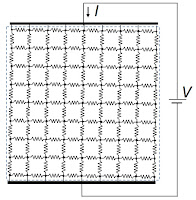The electrical behavior of tissue represented by a grid of resistors.

Now rotate the grid by 45°. In this case, the current takes a tortuous path through the tissue, with the vertical path length increasing by the square root of two. However, more vertical lines are present per unit length in the horizontal direction (count ’em). How many more? The square root of two more! So, the grid has a resistance R. From a microscopic point of view, the conductivity is indeed isotropic.The electrical behavior of tissue represented by a rotated grid of resistors.

Next, replace the resistors by springs. When you pull upwards, the vertical springs stretch with a spring constant k. Using a similar analysis as performed above, the net spring constant of the grid is also k.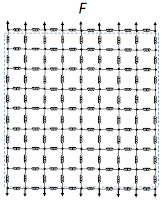The mechanical behavior of tissue represented by a grid of springs.

Now analyze the grid after it's been rotated by 45°. Even if the spring constant were huge (that is, if the springs were very stiff), the grid would stretch by shearing the rotated squares into diamonds. The tissue would have almost no Young’s modulus in the 45° direction and the Poisson's ratio would be about one; the grid would contract horizontally as it expanded vertically (even if the springs themselves didn't stretch at all). This arises because the springs act as if they're connected by hinges. It reminds me of those gates my wife and I installed to prevent our young daughters from falling down the steps. You would need horizontal struts or vertical ties to prevent such shearing.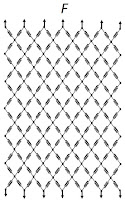The mechanical behavior of tissue represented by a rotated grid of springs.

In conclusion, you can't represent the mechanical behavior of an isotropic tissue using a square grid of springs without struts or ties. Such a microscopic structure corresponds to cloth, which is anisotropic. A square grid fails to capture properly the shearing of the tissue. You can, however, represent the electrical behavior of an isotropic tissue using a square grid of resistors without “electrical struts or ties.”

Gordon elaborated on the anisotropic mechanical properties of cloth in his own engaging way.
In 1922 a dressmaker called Mlle Vionnet set up shop in Paris and proceeded to invent the “bias cut.” Mlle Vionnet had probably never heard of her distinguished compatriot S. D. Poisson—still less of his ratio—but she realized intuitively that there are more ways of getting a fit than by pulling on strings…if the cloth is disposed at 45°…one can exploit the resulting large lateral contraction so as to get a clinging effect.Structures: Or Why Things Don't Fall Down, by J. E. Gordon.